# Test: Electrical & Electronic Measurements- 1

## 20 Questions MCQ Test Mock Test Series for SSC JE Electrical Engineering | Test: Electrical & Electronic Measurements- 1

Description
Attempt Test: Electrical & Electronic Measurements- 1 | 20 questions in 12 minutes | Mock test for SSC preparation | Free important questions MCQ to study Mock Test Series for SSC JE Electrical Engineering for SSC Exam | Download free PDF with solutions
QUESTION: 1

### An electrodynamometer type of instruments finds its major use as

Solution:

Precision type electrodynamometer is used in standardization process of potentiometer.

It is a transfer instrument. A transfer instrument is one which is calibrated with a d.c. source and used without any modifications for a.c. measurements. It has same accuracy for both D.C. and A.C. measurements.

Hence an electrodynamometer type of instruments are used as both standard and transfer instruments.

QUESTION: 2

### An instrument transformer is used to extend the range of-

Solution:

Instrument transformers are of two types.

1. Potential transformer

2. Current transformer

These are used to extend the range of induction type of instruments.

QUESTION: 3

### Perfect reproducibility means the instrument has

Solution:

Reproducibility: It is the degree of closeness with which given value may be repeatedly measured. It may be specified in terms of units for a given period of time.

Perfect reproducibility means that the instrument has no drift.

No drift means that with a given input the measured values do not vary with time.

QUESTION: 4

A wattmeter reads 25.34 W. The absolute error in measurement is -0.11 W. What is the true value of power:

Solution:

Given that,

Measured value = 25.34 W

Absolute error = -0.11 W

Absolute error = Measured value – true value

⇒ -0.11 = 25.34 – true value

⇒ 25.34 + 0.11 = 25.45 W

QUESTION: 5

For wire wound strain gauges, the gauge factor is defined as-

Solution:

The gauge factor is defined as the ratio of per unit change in resistance to per unit change in length.

Gauge factor,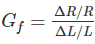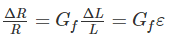Where ε = strain = ΔL/L

The gauge factor can be written as

= Resistance change due to change of length + Resistance change due to change in area + Resistance change due to piezo resistive effect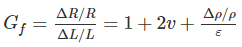If the change in the value of resistivity of a material when strained is neglected, the gauge factor is: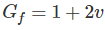The above equation is valid only when Piezo resistive effect that is change in resistivity due to strain is almost neglected.

For wire wound strain gauges, Piezo resistive effect is almost negligible.

QUESTION: 6

Which one of the following types of instruments does suffer from error due to magnetic hysteresis?

Solution:

Hysteresis error in PMMC Instrument: It can be reduced by providing Al frame in the moving system since AL is having a thin hysteresis loop. So that the difference between magnetic fields is less. We can’t eliminate hysteresis error but we can reduce.

Hysteresis error in MI Instrument: Hysteresis error is more in MI instruments. Due to hysteresis effect, the value flux density for the same current, while ascending and descending are different. While descending, the flux density is high and while ascending it is lesser. So we can reduce this error by using small iron parts which can demagnetize quickly.

Hysteresis error in EMMC Instrument: Hysteresis error is absent in EMMC instruments, since there is no iron related material in the moving system.

Order of Hysteresis error in descending order

MI > PMMC > EMMC

QUESTION: 7

For accuracy of the instrument which is necessary

Solution:
QUESTION: 8

A null type of instrument as compared to a deflecting type instrument has

Solution:

The accuracy of null type instruments is higher than that of deflection type. This is because the opposing effect is calibrated with the help of standards which have high degree of accuracy. Accuracy of deflection type instruments is dependent upon their calibration which depends upon the instrument constants which are normally not known to a high degree of accuracy.

In the null type of instruments, the measured quantity is balanced out. This means the detector has to cover a small range around the balance (null) point and therefore can be made highly sensitive. Also the detector need not be calibrated since it has only to detect the presence and direction of unbalance and not the magnitude of unbalance. A deflection type of instrument must be larger in size, more rugged, and thus less sensitive if it is to measure large magnitude of unknown quantity.

Deflection type of instruments have faster response than null type instruments.

QUESTION: 9

A pointer of the instrument once deflected returns to zero position when the current is removed due to:

Solution:

The controlling torque is to control the pointer to a definite value which is proportional to quantity being measured. In absence of controlling torque, the pointer will swing beyond its final steady state position and the deflection will be indefinite. After removal of moving mechanism the pointer has to come back to its initial position, but in absence of controlling torque the pointer won’t come back to its initial position. The following mechanism can be used for producing controlling torque.

The deflecting torque is used for deflection, the controlling torque acts opposite to the deflecting torque. So before coming to rest the pointer always oscillates due to inertia, so to bring the pointer rest within a short time we will use damping torque without effecting controlling torque (or) inertia.

QUESTION: 10

Two 100V Full scale PMMC type dc voltmeter having figure of merit of 10 kΩ/v and 20 kΩ/v are connected series .The series combination can be used to measure a maximum dc voltage of

Solution:

Now IFSD 1=0.1mA ,IFSD2=0.05mA

So,voltmeter 2 reads the full scales current allowed is 0.05mA and and voltmeter one reads half of its full scale value so the total reading is 150V.

QUESTION: 11

The value of resistances in a potential divider arrangement to convert a basic d Arsonval meter movement with an internal resistance of 100 Ω and a full scale current of 1 mA to a multi range DC voltmeter with ranges 0-150 V and 0-300 V are:

Solution:

Given that,

Im = 1 mA

Rm = 100 Ω

Vm = ImRm = 0.1 V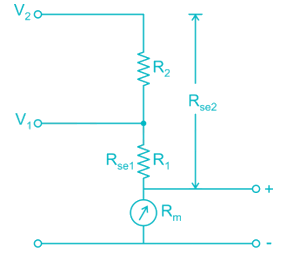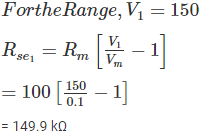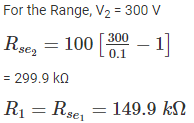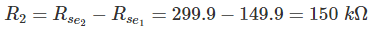QUESTION: 12

The power in a 3 phase circuit is measured with the help of 2 watt meters. The reading of one of watt meters is positive and that of the others is negative. The magnitude of readings is different. It can be concluded that the power factor of the circuit is

Solution:

We know that, power factor is given by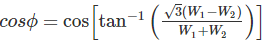Given that,

W1 = +ve value

W2 = -ve value

Here power factor angle varies from 60 degrees to 90 degrees.

Hence power factor varies from zero to 0.5 lagging

QUESTION: 13

The current and potential coils of a dynamometer type wattmeter were accidentally interchanged while connecting. After energizing the circuit, it was observed that the wattmeter did not show the reading, this could be due to:

Solution:

Electrodynamometer instrument has two fixed coils and one moving coil. For using this instrument as a wattmeter to measure the power, the fixed coils acts as a current coil and must be connected in series with a load. The moving coil acts as a pressure coil and must be connected across the supply terminals.

In the question given that, the current and potential coils of a dynamometer type wattmeter were accidentally interchanged while connecting. Hence total voltage applies across current coil which has very less resistance which leads to damage the current coil.

QUESTION: 14

Which of the following is not a type of wattmeter?

Solution:

Moving coil permanent magnet instrument can be used as ammeter and voltmeter but not as wattmeter.

Electrodynamometer instrument has two fixed coils and one moving coil. For using this instrument as a wattmeter to measure the power, the fixed coils act as a current coil and must be connected in series with a load. The moving coil acts as a pressure coil and must be connected across the supply terminals.

Electrostatic watt-meters are used for measurement of small amount of power, practically when the voltage is high and power factor is low. This type of wattmeter is also used for measurement of dielectric loss of cables on alternating voltage and for calibration of watt-meters and energy meters.

QUESTION: 15

The power of 6-phase circuit can be measured with a minimum of:

Solution:

According to Blondel’s theorem the no. of watt-meters to be required to measure the total power in n-phase system is either N (or) (N – 1). When separate neutral wire is available in the system the no. of watt-meters to be required is N. When the neutral wire is not available in the system, then the no. of watt-meters to be required is (N – 1). One line is acts as a common line for return path.

Hence minimum number of watt-meters required = 5

QUESTION: 16

One single phase wattmeter operating on 230 V and 5 A for 5 hours makes 1940 revolutions. Meter constant in revolution is 400. The power factor of the load will be:

Solution:

Given that,

Voltage (V) = 230 V

Current (I) = 5 A

Time (t) = 5 hours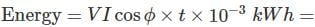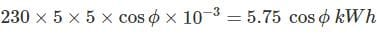Number of revolutions = 1940

Meter constant = 400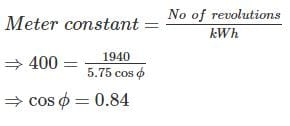QUESTION: 17

A single phase energy meter has a constant of 1200 revolution/kWh. When a load of 200 W is connected, the disc rotates at 4.2 revolutions per min. If the load is on for 10 hours, the meter records an excess of

Solution:

True Kwh = 200 × 10 = 2 kWh

Given that, disc rotates at 4.2 revolutions per minute.

Recorded revolutions = 4.2 × 60 × 10 = 2520

Meter constant = 1200 revolution/kWh

Measured value of kWh = 2520/1200 = 2.1 kW

Excess value of record = measured value – true value = 2.1 – 2 = 0.1 kW

QUESTION: 18

Match List-I (Transducer) with List-2 (Characteristics) and select the correct answer using codes given below the lists: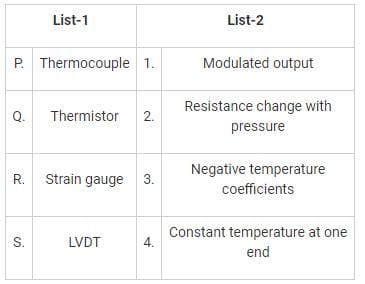Solution:

Thermocouple has constant temperature at one end.

With a negative temperature coefficient thermistor, when the temperature increases the resistance will decreases.

A strain gauge is a sensor whose resistance varies with applied force. It converts force, pressure, tension, weight, etc., into a change in electrical resistance which can then be measured.

Linear variable differential transformer is a common type of electromechanical transducer that can convert the rectilinear motion of an object to which it is coupled mechanically into a corresponding electrical signal.

QUESTION: 19

The purpose of SYNC control in a CRO is to:

Solution:

There must be synchronization between the sweep and the signal being measured. Synchronization is done to produce stationary pattern.

SYNC control in a CRO is used to lock the display of signal.

QUESTION: 20

Which one of the following is a passive transducer?

Solution:

Transducers which require an external power source for their operation is called as a passive transducer. They produce an output signal in the form of some variation in resistance, capacitance or any other electrical parameter, which then has to be converted to an equivalent current or voltage signal. LVDT is an example of passive transducer. LVDT is used as an inductive transducer that convert motion into the electrical signal.Use Code STAYHOME200 and get INR 200 additional OFF Use Coupon Code